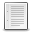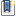File : Details

# Mandelbrot set generator

Draws the Mandebrot set for a list of usual mathematical functions
Details
Version
2.0
Author
Jérôme LABATUT
Maintainer
Jérôme LABATUT
Categories
Supported Scilab Version
5.5
Creation Date
February 26, 2016Description
```            Draws the Mandelbrot set for the following list of mathematical functions :

z -> z^2 + c
z -> z^3 + c
z -> z^4 + c
z -> z^5 + c

z -> c*z*(1 - z)
z -> z^3 - z + c;

z -> 1/2*(z + 1/z) + c
z -> z^2 + 1/z.^2 + c

z -> c*sin(z)
z -> c*cos(z)
z -> c*tan(z)

z -> c*sinh(z)
z -> c*cosh(z)
z -> c*tan(z)

z -> c*exp(z)
z -> c*log(z)
z -> z^z + c

Button "Julia" allows user to click on the Mandelbrot set to select a
value of C whose associated Julia set is drawn.

Likewise button "J-Frontiere" draws iteratively the border of the
selected Julia set, but at the moment only for the classical Mandelbrot set.            ```Files (1)Miscellaneous file
```
```News (0)Comments (0)Leave a comment
Login withEmail notifications